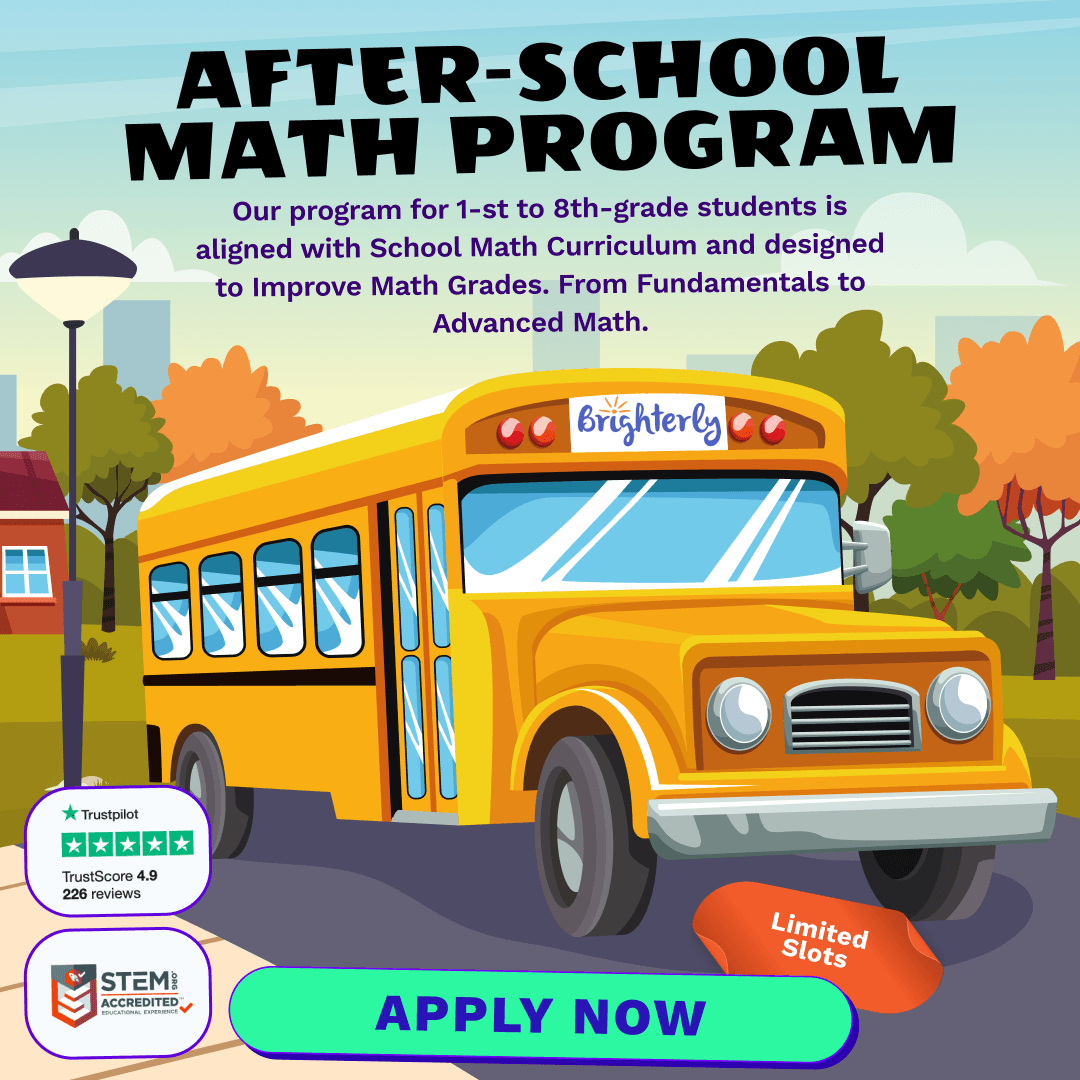# Basic Subtraction Math Practice Test for 2nd Grade – [Easy]

Subtraction is one of the foundational mathematical operations every child learns. By the time they reach the 2nd grade, students should be more comfortable with subtraction, delving deeper into its various nuances. Brighterly, a renowned platform for math for children, offers a comprehensive guide to mastering basic subtraction for the 2nd grade.

## Understanding the Basics

Every math journey begins with the basics. Just as you cannot build a house without a foundation, you cannot master math without understanding subtraction’s core concepts. At its essence, subtraction is the process of taking one number away from another. For instance, if you have five apples and you eat two, you’re left with three. This is represented as `5 - 2 = 3`.

• Subtrahend: The number to be subtracted.
• Minuend: The number from which the subtrahend is to be subtracted.
• Difference: The result of subtracting one number from another.

It’s essential for children to recognize these terms and the process they represent, as they will form the building blocks for more advanced mathematical tasks in the future.

## Techniques and Tips for Effective Subtraction

While the concept of subtraction might seem straightforward, there are strategies that can help students become more proficient. Here are some tried-and-true methods:

1. Counting Backwards: Start with the minuend and count backwards until you’ve subtracted the subtrahend. This is particularly useful for small numbers.
2. Using a Number Line: Visual learners can benefit from drawing a number line and hopping back to find the difference.
3. Regrouping or Borrowing: For more significant numbers, children may need to borrow from the neighboring digit. This method ensures they’re subtracting the correct amounts.

## Conclusion

Subtraction is a pivotal skill in the mathematical journey of every student. With the right techniques, consistent practice, and the invaluable resources from Brighterly, any 2nd grader can become a subtraction superstar. Dive deep into the world of numbers and let your child shine brightly!

Basic Subtraction Practice Test for 2nd Grade

Get ready for math lessons with Brighterly! Designed specifically with budding mathematicians in mind, this easy-level test is perfect for those just starting their subtraction journey. At Brighterly, we believe in laying a strong foundation for a brighter mathematical future.

1 / 20

What is 10 - 5?

2 / 20

Subtract the smallest number from 15:

3 / 20

If you had 8 candies and ate 3, how many would you have left?

4 / 20

12 - 9 = ?

5 / 20

What is 7 - 0?

6 / 20

Subtract 3 from 11:

7 / 20

If there were 20 birds on a tree and 4 flew away, how many are left?

8 / 20

Subtract 10 from 15:

9 / 20

What is 9 - 4?

10 / 20

If you have 14 cookies and give 5 away, how many are left?

11 / 20

18 - 10 = ?

12 / 20

Subtract 6 from 13:

13 / 20

What is 10 - 7?

14 / 20

What is 6 - 2?

15 / 20

Subtract 4 from 9:

16 / 20

If there are 10 dogs in a park and 3 leave, how many are still in the park?

17 / 20

What is 14 - 6?

18 / 20

Subtract 7 from 15:

19 / 20

If you buy 15 toys and give 4 to your friend, how many do you have left?

20 / 20

If there are 16 flowers in a vase and you take 5 away, how many remain?

0%
Poor Level
Weak math proficiency can lead to academic struggles, limited college, and career options, and diminished self-confidence.
Mediocre Level
Weak math proficiency can lead to academic struggles, limited college, and career options, and diminished self-confidence.
Needs Improvement
Start practicing math regularly to avoid your child`s math scores dropping to C or even D.
High Potential
It's important to continue building math proficiency to make sure your child outperforms peers at school.

After-School Math Programs• Our program for 1st to 8th grade students is aligned with School Math Curriculum.

After-School Math Programs
Our program for 1st to 8th grade students is aligned with School Math Curriculum.